# FXOS8700CQ - Bare metal example project

cancel
Showing results for
Did you mean:

# FXOS8700CQ - Bare metal example project

## FXOS8700CQ - Bare metal example project

Hi Everyone,

As I am often asked for a simple bare metal example code for the Xtrinsic FXOS8700CQ 6-axis sensor, I would like to share here one of my examples I have created for this part while working with the Freescale FRDM-KL25Z platform and FRDM-FXS-MULTI(-B) sensor expansion board that features many of the Xtrinsic sensors including the FXOS8700CQ. The FreeMASTER tool is used to visualize both the acceleration and magnetic data that are read from the FXOS8700CQ using an interrupt technique through the I2C interface.

This example illustrates:

1. Initialization of the MKL25Z128 MCU (mainly I2C and PORT modules).

2. I2C data write and read operations.

3. Initialization of the FXOS8700CQ.

4. Simple accelerometer offset calibration based on the AN4069.

5. Simple magnetic hard-iron offset calibration.

6. Output data reading using an interrupt technique.

7. Conversion of the output values from registers 0x01 – 0x06 and 0x33 – 0x38 to real values in g’s and µT and simple heading angle calculation.

8. Visualization of the calculated values in the FreeMASTER tool.

1. As you can see in the FRDM-FXS-MULTI(-B)/FRDM-KL25Z schematics and the image below, I2C signals are routed to the I2C1 module (PTC1 and PTC2 pins) of the KL25Z MCU and the INT1 output is connected to the PTD4 pin (make sure that pins 1-2 of J3 on the sensor expansion board are connected together using a jumper). The INT1 output of the FXOS8700CQ is configured as a push-pull active-low output, so the corresponding PTD4 pin configuration is GPIO with an interrupt on falling edge.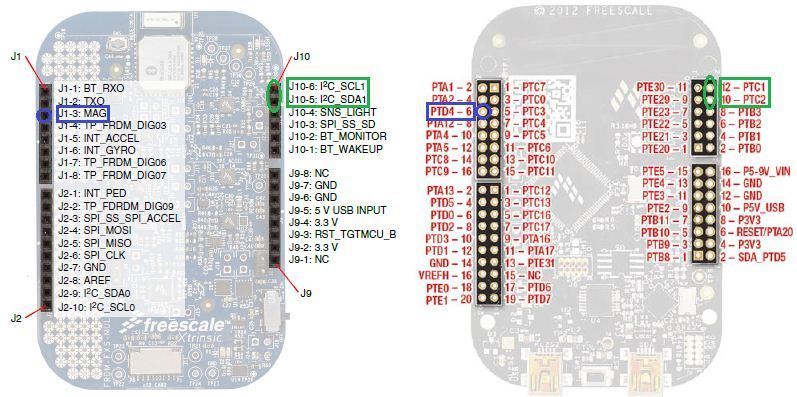The MCU is, therefore, configured as follows.

void MCU_Init(void)

{

//I2C1 module initialization

SIM_SCGC4 |= SIM_SCGC4_I2C1_MASK;        // Turn on clock to I2C1 module

SIM_SCGC5 |= SIM_SCGC5_PORTC_MASK;       // Turn on clock to Port C module

PORTC_PCR1 |= PORT_PCR_MUX(0x2);         // PTC1 pin is I2C1 SCL line

PORTC_PCR2 |= PORT_PCR_MUX(0x2);         // PTC2 pin is I2C1 SDA line

I2C1_F  |= I2C_F_ICR(0x14);              // SDA hold time = 2.125us, SCL start hold time = 4.25us, SCL stop hold time = 5.125us

I2C1_C1 |= I2C_C1_IICEN_MASK;            // Enable I2C0 module

//Configure the PTD4 pin (connected to the INT1 of the FXOS8700CQ) for falling edge interrupts

SIM_SCGC5 |= SIM_SCGC5_PORTD_MASK;       // Turn on clock to Port A module

PORTD_PCR4 |= (0|PORT_PCR_ISF_MASK|      // Clear the interrupt flag

PORT_PCR_MUX(0x1)|       // PTD4 is configured as GPIO

PORT_PCR_IRQC(0xA));     // PTD4 is configured for falling edge interrupts

//Enable PORTD interrupt on NVIC

NVIC_ICPR |= 1 << ((INT_PORTD - 16)%32);

NVIC_ISER |= 1 << ((INT_PORTD - 16)%32);

}

2. The 7-bit I2C address of the FXOS8700CQ is 0x1E since both SA0 and SA1 lines are shorted to GND using jumpers J21 and J23 on the sensor board. As shown above, the SCL line is connected to the PTC1 pin and SDA line to the PTC2 pin. The I2C clock frequency is 125 kHz.

The screenshot below shows the write operation which writes the value 0x35 to the CTRL_REG1 (0x2A).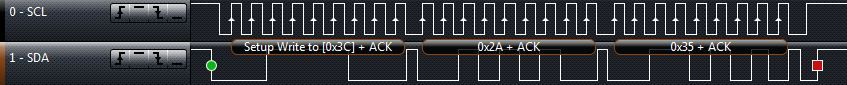And here is the single byte read from the WHO_AM_I register 0x0D. As you can see, it returns the correct value 0xC7.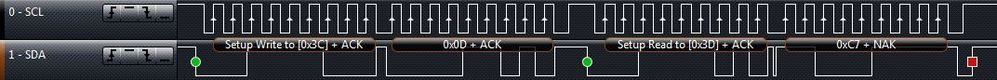Multiple bytes of data can be read from sequential registers after each FXOS8700CQ acknowledgment (AK) is received until a no acknowledge (NAK) occurs from the KL25Z followed by a stop condition (SP) signaling an end of transmission. A burst read of 6 bytes from registers 0x33 to 0x38, that is performed in the calibration routine “FXOS8700CQ_Mag_Calibration()”, is shown below. It also shows when the INT1 pin is automatically cleared.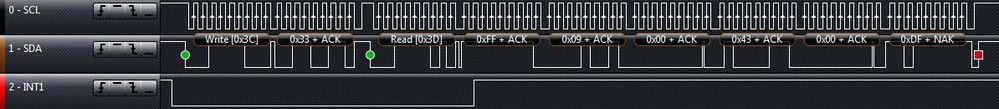3. At the beginning of the initialization, all registers are reset to their default values by setting the RST bit of the CTRL_REG2 register. Then the FXOS8700CQ is initialized as shown below.

void FXOS8700CQ_Init (void)

{

I2C_WriteRegister(FXOS8700CQ_I2C_ADDRESS, CTRL_REG2, 0x40);          // Reset all registers to POR values

Pause(0x631);        // ~1ms delay

I2C_WriteRegister(FXOS8700CQ_I2C_ADDRESS, XYZ_DATA_CFG_REG, 0x00);   // +/-2g range with 0.244mg/LSB

I2C_WriteRegister(FXOS8700CQ_I2C_ADDRESS, M_CTRL_REG1, 0x1F);        // Hybrid mode (accelerometer + magnetometer), max OSR

I2C_WriteRegister(FXOS8700CQ_I2C_ADDRESS, M_CTRL_REG2, 0x20);        // M_OUT_X_MSB register 0x33 follows the OUT_Z_LSB register 0x06 (used for burst read)

I2C_WriteRegister(FXOS8700CQ_I2C_ADDRESS, CTRL_REG2, 0x02);          // High Resolution mode

I2C_WriteRegister(FXOS8700CQ_I2C_ADDRESS, CTRL_REG3, 0x00);          // Push-pull, active low interrupt

I2C_WriteRegister(FXOS8700CQ_I2C_ADDRESS, CTRL_REG4, 0x01);          // Enable DRDY interrupt

I2C_WriteRegister(FXOS8700CQ_I2C_ADDRESS, CTRL_REG5, 0x01);          // DRDY interrupt routed to INT1 - PTD4

I2C_WriteRegister(FXOS8700CQ_I2C_ADDRESS, CTRL_REG1, 0x35);          // ODR = 3.125Hz, Reduced noise, Active mode

}

4. A simple accelerometer offset calibration method is implemented according to the AN4069.

void FXOS8700CQ_Accel_Calibration (void)

{

char X_Accel_offset, Y_Accel_offset, Z_Accel_offset;

I2C_WriteRegister(FXOS8700CQ_I2C_ADDRESS, CTRL_REG1, 0x00);          // Standby mode

Xout_Accel_14_bit = ((short) (AccelMagData<<8 | AccelMagData)) >> 2;        // Compute 14-bit X-axis acceleration output value

Yout_Accel_14_bit = ((short) (AccelMagData<<8 | AccelMagData)) >> 2;        // Compute 14-bit Y-axis acceleration output value

Zout_Accel_14_bit = ((short) (AccelMagData<<8 | AccelMagData)) >> 2;        // Compute 14-bit Z-axis acceleration output value

X_Accel_offset = Xout_Accel_14_bit / 8 * (-1);         // Compute X-axis offset correction value

Y_Accel_offset = Yout_Accel_14_bit / 8 * (-1);         // Compute Y-axis offset correction value

Z_Accel_offset = (Zout_Accel_14_bit - SENSITIVITY_2G) / 8 * (-1);          // Compute Z-axis offset correction value

I2C_WriteRegister(FXOS8700CQ_I2C_ADDRESS, CTRL_REG1, 0x35);          // Active mode again

}

5. A simple software calibration of magnetic hard-iron offset consists of recording the minimum and maximum magnetometer readings while rotating the board horizontally and vertically and then computing the calibration values from their average. The calibration time is defined by the number of samples taken during calibration (local variable “i” in the while loop) and selected ODR. In my example I use 94 samples at 3.125Hz, so the calibration routine takes 30s.

void FXOS8700CQ_Mag_Calibration (void)

{

short Xout_Mag_16_bit_avg, Yout_Mag_16_bit_avg, Zout_Mag_16_bit_avg;

short Xout_Mag_16_bit_max, Yout_Mag_16_bit_max, Zout_Mag_16_bit_max;

short Xout_Mag_16_bit_min, Yout_Mag_16_bit_min, Zout_Mag_16_bit_min;

char i = 0;

while (i < 94)             // This takes ~30s (94 samples * 1/3.125)

{

{

Xout_Mag_16_bit = (short) (AccelMagData<<8 | AccelMagData);        // Compute 16-bit X-axis magnetic output value

Yout_Mag_16_bit = (short) (AccelMagData<<8 | AccelMagData);        // Compute 16-bit Y-axis magnetic output value

Zout_Mag_16_bit = (short) (AccelMagData<<8 | AccelMagData);        // Compute 16-bit Z-axis magnetic output value

// Assign first sample to maximum and minimum values

if (i == 0)

{

Xout_Mag_16_bit_max = Xout_Mag_16_bit;

Xout_Mag_16_bit_min = Xout_Mag_16_bit;

Yout_Mag_16_bit_max = Yout_Mag_16_bit;

Yout_Mag_16_bit_min = Yout_Mag_16_bit;

Zout_Mag_16_bit_max = Zout_Mag_16_bit;

Zout_Mag_16_bit_min = Zout_Mag_16_bit;

}

// Check to see if current sample is the maximum or minimum X-axis value

if (Xout_Mag_16_bit > Xout_Mag_16_bit_max)    {Xout_Mag_16_bit_max = Xout_Mag_16_bit;}

if (Xout_Mag_16_bit < Xout_Mag_16_bit_min)    {Xout_Mag_16_bit_min = Xout_Mag_16_bit;}

// Check to see if current sample is the maximum or minimum Y-axis value

if (Yout_Mag_16_bit > Yout_Mag_16_bit_max)    {Yout_Mag_16_bit_max = Yout_Mag_16_bit;}

if (Yout_Mag_16_bit < Yout_Mag_16_bit_min)    {Yout_Mag_16_bit_min = Yout_Mag_16_bit;}

// Check to see if current sample is the maximum or minimum Z-axis value

if (Zout_Mag_16_bit > Zout_Mag_16_bit_max)    {Zout_Mag_16_bit_max = Zout_Mag_16_bit;}

if (Zout_Mag_16_bit < Zout_Mag_16_bit_min)    {Zout_Mag_16_bit_min = Zout_Mag_16_bit;}

i++;

}

}

Xout_Mag_16_bit_avg = (Xout_Mag_16_bit_max + Xout_Mag_16_bit_min) / 2;            // X-axis hard-iron offset

Yout_Mag_16_bit_avg = (Yout_Mag_16_bit_max + Yout_Mag_16_bit_min) / 2;            // Y-axis hard-iron offset

Zout_Mag_16_bit_avg = (Zout_Mag_16_bit_max + Zout_Mag_16_bit_min) / 2;            // Z-axis hard-iron offset

// Left-shift by one as magnetometer offset registers are 15-bit only, left justified

Xout_Mag_16_bit_avg <<= 1;

Yout_Mag_16_bit_avg <<= 1;

Zout_Mag_16_bit_avg <<= 1;

I2C_WriteRegister(FXOS8700CQ_I2C_ADDRESS, CTRL_REG1, 0x00);          // Standby mode to allow writing to the offset registers

I2C_WriteRegister(FXOS8700CQ_I2C_ADDRESS, MOFF_X_LSB_REG, (char) (Xout_Mag_16_bit_avg & 0xFF));

I2C_WriteRegister(FXOS8700CQ_I2C_ADDRESS, MOFF_X_MSB_REG, (char) ((Xout_Mag_16_bit_avg >> & 0xFF));

I2C_WriteRegister(FXOS8700CQ_I2C_ADDRESS, MOFF_Y_LSB_REG, (char) (Yout_Mag_16_bit_avg & 0xFF));

I2C_WriteRegister(FXOS8700CQ_I2C_ADDRESS, MOFF_Y_MSB_REG, (char) ((Yout_Mag_16_bit_avg >> & 0xFF));

I2C_WriteRegister(FXOS8700CQ_I2C_ADDRESS, MOFF_Z_LSB_REG, (char) (Zout_Mag_16_bit_avg & 0xFF));

I2C_WriteRegister(FXOS8700CQ_I2C_ADDRESS, MOFF_Z_MSB_REG, (char) ((Zout_Mag_16_bit_avg >> & 0xFF));

I2C_WriteRegister(FXOS8700CQ_I2C_ADDRESS, CTRL_REG1, 0x35);          // Active mode again

}

6. In the ISR, only the interrupt flag is cleared and the DataReady variable is set to indicate the arrival of new data.

void PORTD_IRQHandler()

{

PORTD_PCR4 |= PORT_PCR_ISF_MASK;                // Clear the interrupt flag

}

7. The output values from accelerometer registers 0x01 – 0x06 are first converted to signed 14-bit integer values and afterwards to real values in g’s. Similarly, the output values from magnetometer registers 0x33 – 0x38 are first converted to signed 16-bit integer values and afterwards to real values in microtesla (µT).

If the board remains flat, then the compass heading can be simply computed from the arctangent of the ratio of the two horizontal magnetic field components. I have used the atan2 function which returns the result in radians (-π and π), so I multiply it by 180/π to end up with degrees.

If you are interested in more complex algorithms for a tilt-compensated e-compass with soft-iron calibration, please refer to our eCompass software.

{

// 14-bit accelerometer data

Xout_Accel_14_bit = ((short) (AccelMagData<<8 | AccelMagData)) >> 2;        // Compute 14-bit X-axis acceleration output value

Yout_Accel_14_bit = ((short) (AccelMagData<<8 | AccelMagData)) >> 2;        // Compute 14-bit Y-axis acceleration output value

Zout_Accel_14_bit = ((short) (AccelMagData<<8 | AccelMagData)) >> 2;        // Compute 14-bit Z-axis acceleration output value

// Accelerometer data converted to g's

Xout_g = ((float) Xout_Accel_14_bit) / SENSITIVITY_2G;        // Compute X-axis output value in g's

Yout_g = ((float) Yout_Accel_14_bit) / SENSITIVITY_2G;        // Compute Y-axis output value in g's

Zout_g = ((float) Zout_Accel_14_bit) / SENSITIVITY_2G;        // Compute Z-axis output value in g's

// 16-bit magnetometer data

Xout_Mag_16_bit = (short) (AccelMagData<<8 | AccelMagData);          // Compute 16-bit X-axis magnetic output value

Yout_Mag_16_bit = (short) (AccelMagData<<8 | AccelMagData);          // Compute 16-bit Y-axis magnetic output value

Zout_Mag_16_bit = (short) (AccelMagData<<8 | AccelMagData);        // Compute 16-bit Z-axis magnetic output value

// Magnetometer data converted to microteslas

Xout_uT = (float) (Xout_Mag_16_bit) / SENSITIVITY_MAG;        // Compute X-axis output magnetic value in uT

Yout_uT = (float) (Yout_Mag_16_bit) / SENSITIVITY_MAG;        // Compute Y-axis output magnetic value in uT

Zout_uT = (float) (Zout_Mag_16_bit) / SENSITIVITY_MAG;        // Compute Z-axis output magnetic value in uT

Heading = atan2 (Yout_uT, Xout_uT) * 180 / PI;         // Compute Yaw angle

}

8. The calculated values can be watched in the "Variables" window on the top right of the Debug perspective or in the FreeMASTER application. To open and run the FreeMASTER project, install the FreeMASTER 1.4 application and FreeMASTER Communication Driver.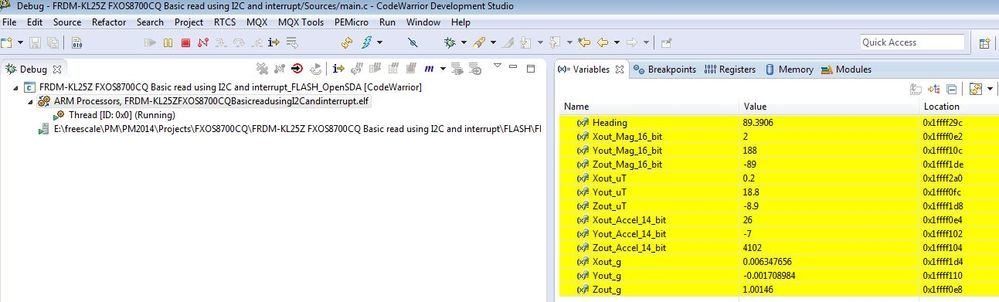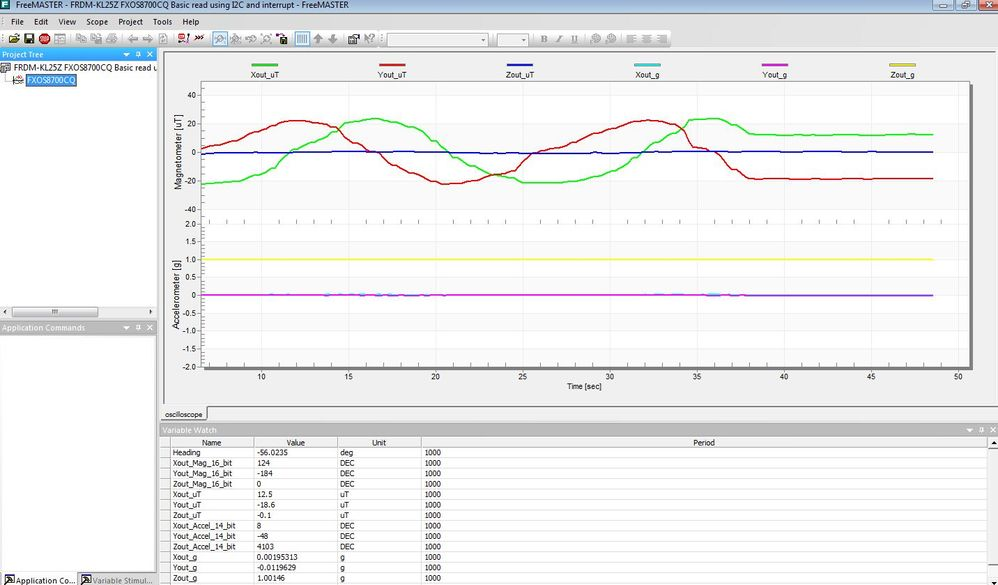Attached you can find the complete source code written in the CW for MCU's v10.5 including the FreeMASTER project.

If there are any questions regarding this simple application, please feel free to ask below. Your feedback or suggestions are also welcome.

Regards,

Tomas

Original Attachment has been moved to: FRDM-KL25Z-FXOS8700CQ-Basic-read-using-I2C-and-interrupt.zip

Original Attachment has been moved to: FreeMASTER---FRDM-KL25Z-FXOS8700CQ-Basic-read-using-I2C-and-interrupt.zip

Labels (2)

• ### Magnetic Sensors

Hi Tomas,

Thanks for posting this project! I want to make a similar project using the FXOS8700CQ that is on the FRDM K64F board and with MQX. Do you know if somebody has done this?

Thanks,
David

Hi David,

Please try contacting Mike Stanley (Mike.Stanley@freescale.com), he and his team have been working on the Xtrinsic sensor fusion library for Kinetis MCUs that is based on MQX Lite RTOS and they recently added support for the FRDM-K64F board.

Regards,

Tomas

Hello,

I am new to these devices. I have been given the task of setting up the FXOS8700CQ on one of our projects. I only intend on using the magnetometer side of the device. I would like to use the interrupt to update a funcation when data is available so it can go and get some data.

If i am using the magnetometer side only, what registers do i need to configure. How do i do a calibration of the magnetometer and general reading of the data from the magnetometer?

Regards,

Luke

Hi Luke,

Regards,

Tomas

Hi David;

Any chance that you got that to work?
I am working on a graduate project and need the same K64f board to work.

Thanks :smileyhappy:

Hi Mustafa,

Have a look at this example code or this post. Hope it helps.

Regards,

Tomas

Hi Tomas,

With the real g’s and µT data, is there an algorithm to calculate the real position?

Thanks,

Tommy

Hi Tommy,

What is the values for SENSITIVITY_MAG=??? & SENSITIVITY_2G=??

Regards,

anil

Hi Thomas,

What is the values for SENSITIVITY_MAG=??? & SENSITIVITY_2G=??

SENSITIVITY_2G = 0.244 (mg)

SENSITIVITY_MAG = 0.1 (uT)

applying the above values in the code. Is it right? or any other values need to specify.

Regards,

anil

Hi Anil,

This is defined in the FXOS8700CQ.h file (Project_Headers/FXOS8700CQ.h).

//FXOS8700CQ Sensitivity

#define SENSITIVITY_2G 4096
#define SENSITIVITY_4G 2048
#define SENSITIVITY_8G 1024
#define SENSITIVITY_MAG 10

Regards,

Tomas

Hi Thomas,

After converted the Raw values and getting the heading angle shows in between -180 to +180.

How to covert from -180 to +180  to 0 to 360 angle.

Regards,

Anil

Hi Anil,

I believe it should be pretty simple:

Heading = 180 + atan2 (Yout_uT, Xout_uT) * 180 / PI;

Regards,

Tomas

Hello,

In accelerometer calibration on 2g sensitivity is used this equation:

Z_Accel_offset = (Zout_Accel_14_bit - SENSITIVITY_2G) / 8 * (-1);

My question is, and when do I use sensitivity 4g and 8g?
Remains the same equation? The number 8, which divided the first part of the equation, is always this value, either for 2g, 4g or 8g?

In my project, I need change the sensitivity and I want calibrate for this new sensitivity.

Thank for help.

Regards.

Alexandre

Hi Alexandre,

SENSITIVITY_2G corresponds to fs[1:0] = 0b00, SENSITIVITY_4G to fs[1:0] = 0b01 and SENSITIVITY_8G to fs[1:0] = 0b10.

Note that the resolution of OFF_X(YZ) registers is 2mg per LSB, that is why I use the number 8 for 2g. For 4g the number is 4 and for 8g it is 2. For more information please refer to the AN4069.

Regards,

Tomas

Hi Tomas,

I'm a greenhand to these devises, I'm try to apply the accelerometer FXOS8700CQ in FRDM-K66F board. I'd like to learn to  how to activate the FXOS8700CQ sensor in FRDM-K66F. May I know where can I get some learning materials such as example codes.

Regards,

Yue.

Version history
Revision #:
2 of 2
Last update:
a week ago
Updated by:Contributors